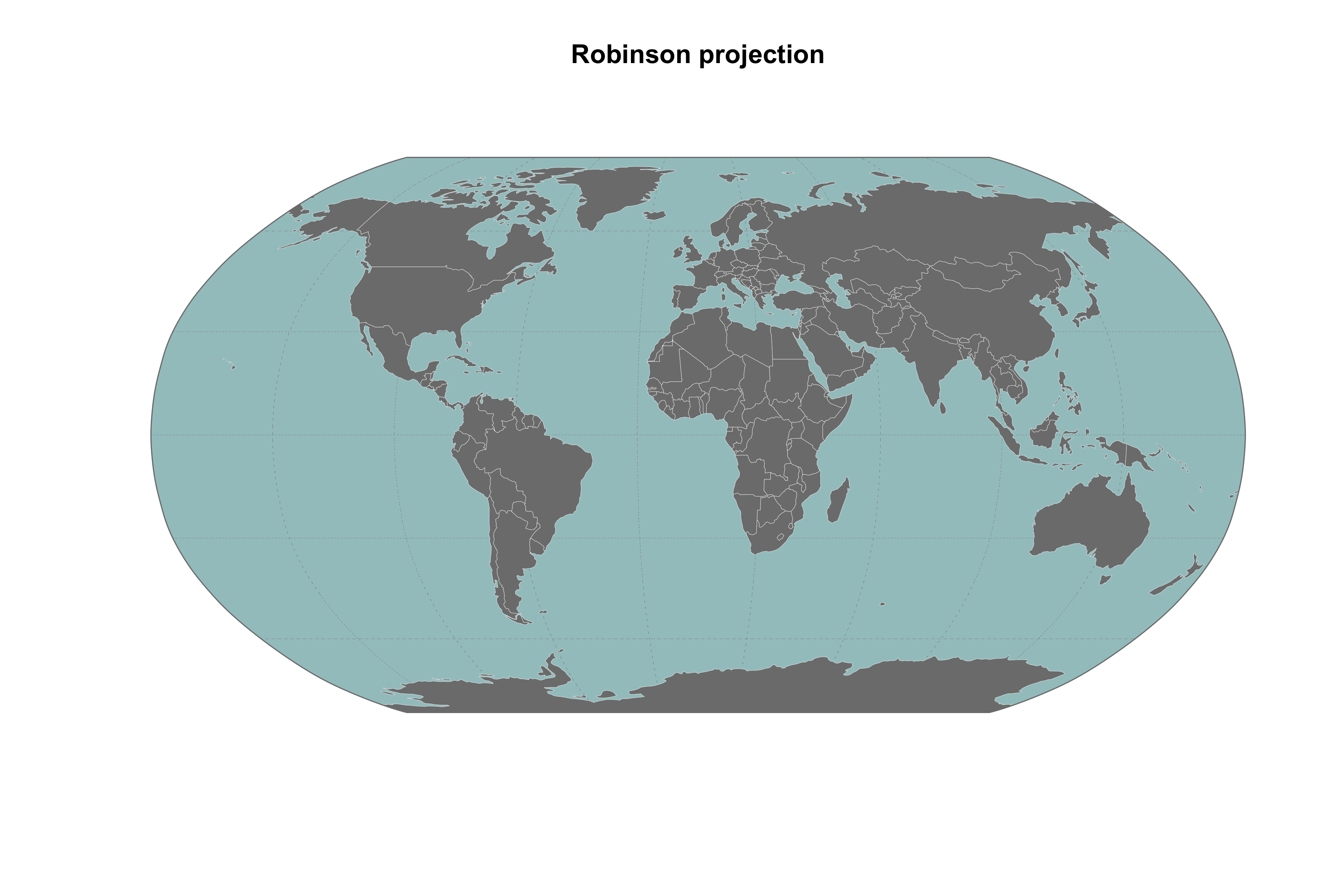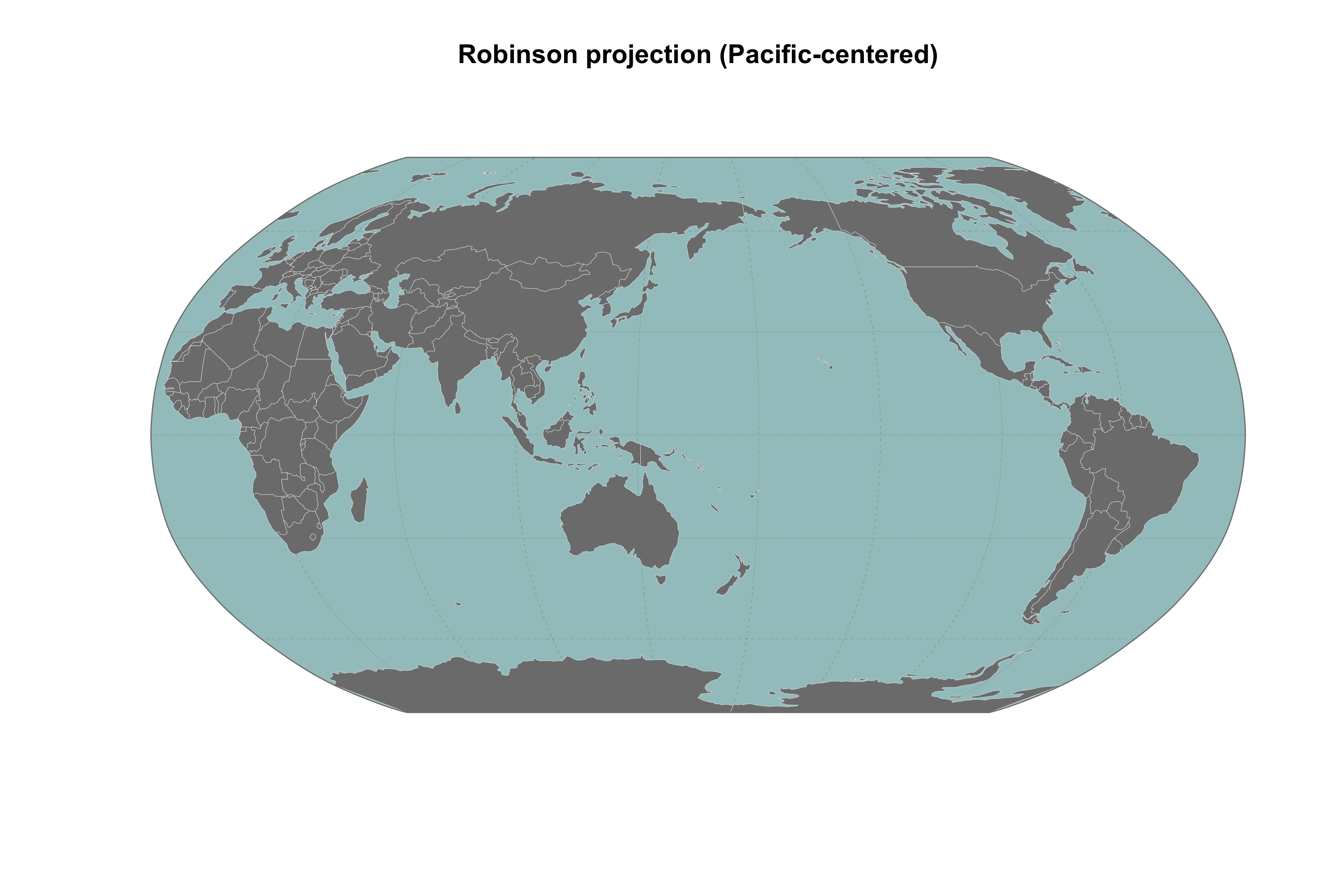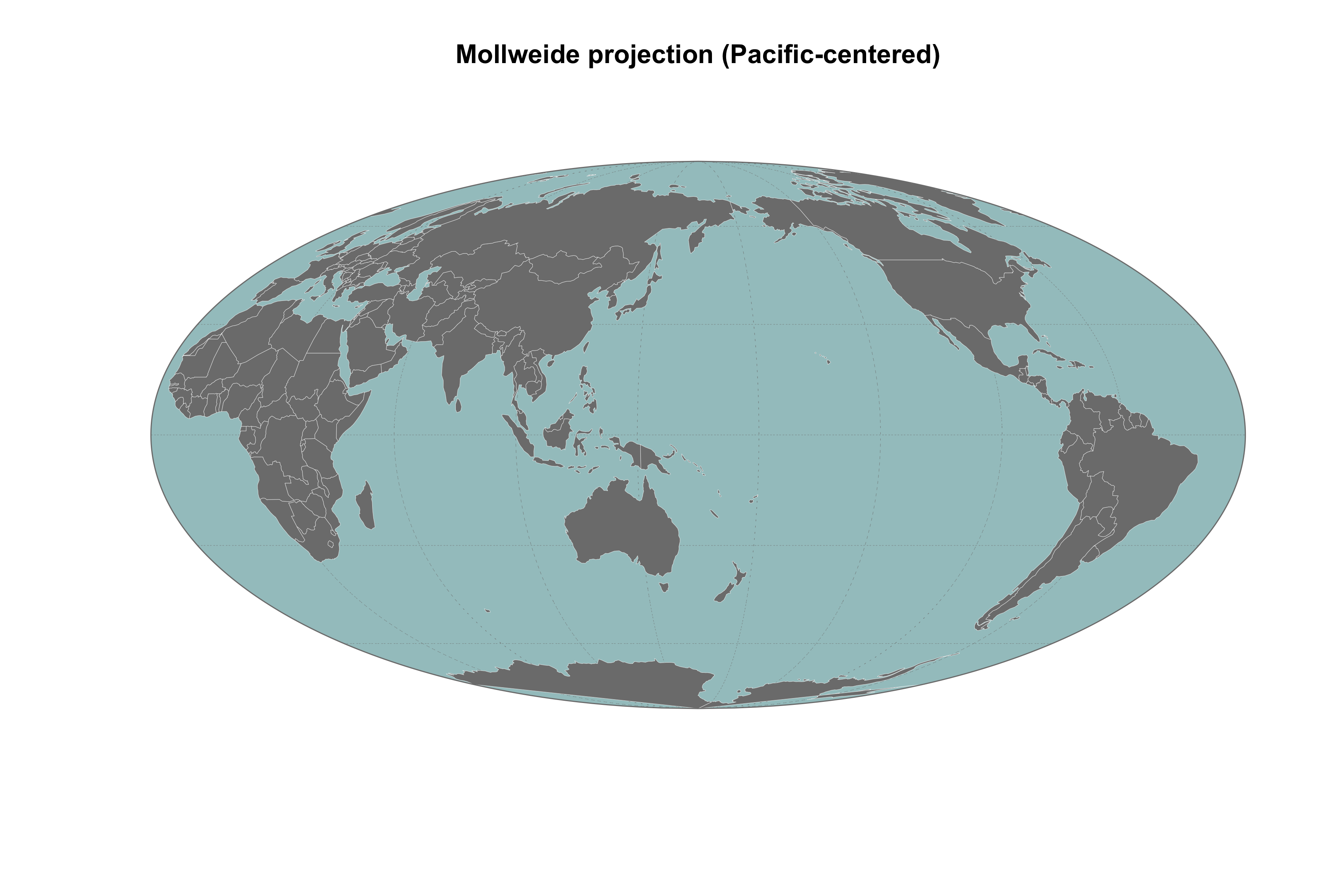The goal of the R package robinmap is to map World countries in the Robinson projection system (or others CRS) and to center the map on a different meridian than Greenwich. It uses the Natural Earth layer as a base map.### System Requirements

This package handles spatial objects and requires some system dependencies (GDAL, PROJ and GEOS). Please visit this page to correctly install these tools.

## Installation

You can install the development version from GitHub with:

# install.packages("remotes")
remotes::install_github("FRBCesab/robinmap")

Then you can attach the package robinmap:

library("robinmap")

## Usage

## Default map ----
robinmap()

## Robinson projection - Pacific-centered ----
robinmap(center = 160)

## World Eckert IV projection - Pacific-centered ----
eckert <- "+proj=eck4 +lon_0=0 +x_0=0 +y_0=0 +ellps=WGS84 +datum=WGS84 +units=m +no_defs"
robinmap(center = 160, crs = eckert)

## Mollweide projection - Pacific-centered ----
mollweide <- "+proj=moll +lon_0=0 +x_0=0 +y_0=0 +datum=WGS84 +units=m +no_defs"
robinmap(center = 160, crs = mollweide)

## Behrmann projection - Pacific-centered ----
behrmann <- "+proj=cea +lon_0=0 +lat_ts=30 +x_0=0 +y_0=0 +datum=WGS84 +ellps=WGS84 +units=m +no_defs"
robinmap(center = 160, crs = behrmann)

## Citation

Please note that the robinmap project is released with a Contributor Code of Conduct. By contributing to this project, you agree to abide by its terms.